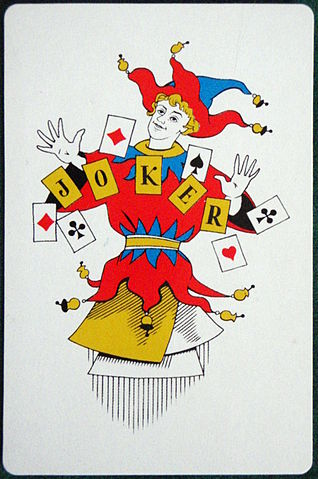# Expectations Between Jokers

Probability Level 5A standard $52$-card deck with $n$ extra jokers (where $n\ge 2$) is shuffled. Given the expected number of cards between the first joker and the last joker exclusive is a positive integer $E$, find the sum of all possible values of $E$.

Details and Assumptions

The $52$-card deck with $n$ extra jokers implies that there are $52+n$ cards in total.

×

Problem Loading...

Note Loading...

Set Loading...